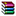# DHSVM结果文件处理程序

DHSVM的一些水文成分（比如降雨蒸散发等）是输出在Mass.Balance这个文件中，按照模拟的时间步长输出的。%% This Code is used to sumarized the default output of DHSVM (Mass.Balance)

%  Initally created by LING ZHANG, zhanglingky@lzb.ac.cn

%  The result is in Excel format; note:the Begining day should be 01/01 if

%  you want to summarize yearly data

%% The meaning for each colunmn

% Total runoff

% Total amount of water in the canopy

% Total amount of water in the soil

% Total amount of snow water equivalent

% Total amount of saturated subsurface flow

% Total amount of water intercepted by channels

% Total amount of water intercepted by roads

% Total amount of water returned by culverts to the land surface

% Total amount of evapotranspiration

% Total amount of precipitation

% Total amount of sublimation from snow on the ground

% Total amount of sublimation from snow in the canopy

% Total amount of water during the previous time step

% Total amount of flow from culverts to the channel

% Total amount of surface flow to the channel

% Total mass balance error for the current time step

%****************** Only the following items will be output*********%

% Total amount of saturated subsurface flow

% Total amount of water intercepted by channels

% Total amount of evapotranspiration

% Total amount of precipitation

% Total amount of surface flow to the channel

%% User difined Parmaters：需要用户自定义！

clc;

clear;

Model_start_year = 1992;

Model_end_year = 1995;

Model_time_step = 3;BalanceSum.rar